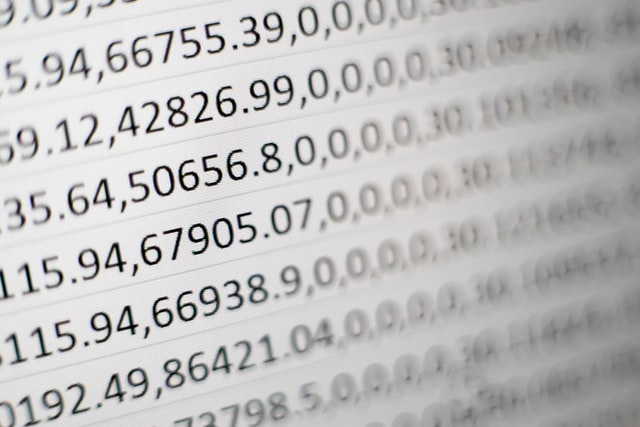## Decimal Expansion of Rational Numbers Class 10th

Introduction We have already studied that the number which can be written in the form of p/q is called a Rational Number. Where p and q are coprime numbers and q ≠ 0. The decimal expansion of rational numbers is either a terminating decimal expansion or a non-terminating repeating (recurring) decimal expansion. In this section, …